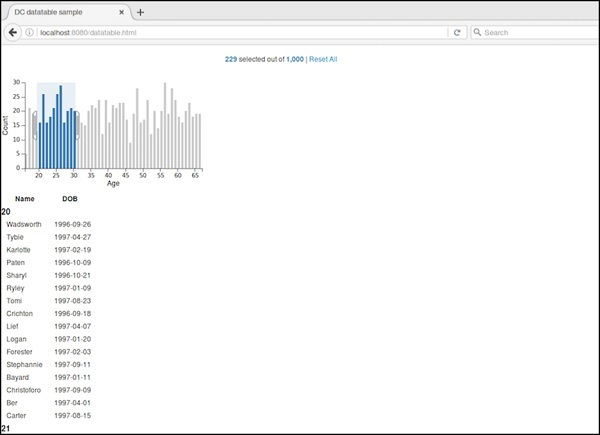# DC.js - Data Table

#### Complete Python Prime Pack for 2023

9 Courses     2 eBooks

#### Artificial Intelligence & Machine Learning Prime Pack

6 Courses     1 eBooks

#### Java Prime Pack 2023

8 Courses     2 eBooks

Data table is used to display the records in a tabular format. It lists out the crossfilter dataset records as explained in this chapter in detail.

## Data Table Methods

Before moving on to draw a data table, we should understand the dc.dataTable class and its methods. It uses a mixin to get the basic functionality of drawing a data table chart, which is defined below −

• dc.baseMixin

The dc.dataTable gets all the methods of this mixin and has its own methods to draw the data table, which are explained as follows.

### beginSlice( [slice])

This method is used to get or set the index of the beginning slice. This method is useful when implementing pagination.

Similarly, you can perform the endSlice() function as well.

### columns( [columns])

This method is used to get or set the column functions. It uses the following method to specify the columns to display.

```chart.columns([
function(d) { return d.mark; },
function(d) { return d.low; },
function(d) { return d.high; },
function(d) { return numberFormat(d.high - d.low); },
function(d) { return d.volume; }
]);
```

Here, d represents a row in the data set. We can use HTML to display columns in the data table.

### group(groupFunction)

This method is used to perform the group function for the data table.

### order( [order])

It is used to sort the order function. If the order is ascending, then the dimension() will use .bottom() to fetch the data, otherwise it will use dimension().top().

## Data Table Example

Let us make a data table in DC. To do this, we need to follow the steps given below −

Let us add styles in the CSS using the coding given below −

```.dc-chart { font-size: 12px; }
.dc-table-group { padding-left: 10px; font-size: 14px; font-weight: bold; }
.dc-table-column { padding-left: 10px; font-size: 12px; font-weight: normal; }
```

Here, we have assigned styles for the chart, table-group and the grid-column.

### Step 2: Create a variable

Let us create a variable in DC as shown below −

```var barChart = dc.barChart('#line'); //
var countChart = dc.dataCount("#mystats");
var tableChart = dc.dataTable("#mytable");
```

Here, we have assigned a barChart variable id in line, countChart id is mystats and the tableChart id is mytable.

### Step 3: Read the data

Read the data from the people.csv file as shown below −

```d3.csv("data/people.csv", function(errors, people) {
var mycrossfilter = crossfilter(people);
}
```

If the data is not present, then it returns an error. Now, assign the data to a crossfilter. Here, we have used the same people.csv file, which was used in our previous charting examples.

It looks like as follows −

```id,name,gender,DOB,MaritalStatus,CreditCardType
1,Damaris,Female,1973-02-18,false,visa-electron
2,Barbe,Female,1969-04-10,true,americanexpress
3,Belia,Female,1960-04-16,false,maestro
4,Leoline,Female,1995-01-19,true,bankcard
5,Valentine,Female,1992-04-16,false,
6,Rosanne,Female,1985-01-05,true,bankcard
7,Shalna,Female,1956-11-01,false,jcb
8,Mordy,Male,1990-03-27,true,china-unionpay

..........................................
..........................................
```

### Step 4: Set the dimension

You can set the dimension using the coding given below −

```var ageDimension = mycrossfilter.dimension(function(data) {
return ~~((Date.now() - new Date(data.DOB)) / (31557600000))
});
```

After the dimension has been assigned, group the age using the coding given below −

```var ageGroup = ageDimension.group().reduceCount();
```

### Step 5: Generate a chart

Now, generate a bar chart using the coding given below −

```barChart
.width(400)
.height(200)
.x(d3.scale.linear().domain([15,70]))
.yAxisLabel("Count")
.xAxisLabel("Age")
.elasticY(true)
.elasticX(true)
.dimension(ageDimension)
.group(ageGroup);
```

Here,

• We have assigned the chart width as 400 and height as 200.
• Next, we have specified the domain range as [15,70].
• We have set the x-axis label as age and y-axis label as count.
• We have specified the elasticY and X function as true.

### Step 6: Create the data table

Now, create the data table using the coding given below −

```countChart
.dimension(mycrossfilter)
.group(mycrossfilter.groupAll());

tableChart
.dimension(ageDimension)
.group(function (data) {
return ~~((Date.now() - new Date(data.DOB)) / (31557600000));
})
```

Here, we have specified the age dimension and group the data.

### Step 7: Render the table

Now, render the grid using the coding below −

```.size(Infinity)
.columns(['name', 'DOB'])
.sortBy(function (d) {
return d.value;
})
.order(d3.ascending);

barChart.render();
countChart.render();
tableChart.render();
```

Here, we have sorted the columns using the DOB and sort the records.

### Step 8: Working example

The complete code is as follows. Create a web page datatable.html and add the following changes to it.

```<html>
<title>DC datatable sample</title>
<link rel = "stylesheet" type = "text/css" href = "css/bootstrap.css">
<link rel = "stylesheet" type = "text/css" href = "css/dc.css"/>

<style>
.dc-chart { font-size: 12px; }
.dc-table-group { padding-left: 10px; font-size: 14px; font-weight: bold; }
.dc-table-column { padding-left: 10px; font-size: 12px; font-weight: normal; }
</style>

<script src = "js/d3.js"></script>
<script src = "js/crossfilter.js"></script>
<script src = "js/dc.js"></script>

<body>
<div>
<div style = "width: 600px;">
<div id = "mystats" class = "dc-data-count" style = "float: right">
<span class = "filter-count"></span> selected out of <span
class = "total-count"></span> | <a href = "javascript:dc.filterAll();
dc.renderAll();">Reset All</a>
</div>
</div>

<div style = "clear: both; padding-top: 20px;">
<div>
<div id = "line"></div>
</div>
</div>

<div style = "clear: both">
<div id = "mytable"></div>
</div>
</div>

<script language = "javascript">
var barChart = dc.barChart('#line'); // , 'myChartGroup');
var countChart = dc.dataCount("#mystats");
var tableChart = dc.dataTable("#mytable");

d3.csv("data/people.csv", function(errors, people) {
var mycrossfilter = crossfilter(people);

// age dimension
var ageDimension = mycrossfilter.dimension(function(data) {
return ~~((Date.now() - new Date(data.DOB)) / (31557600000))
});
var ageGroup = ageDimension.group().reduceCount();

barChart
.width(400)
.height(200)
.x(d3.scale.linear().domain([15,70]))
.yAxisLabel("Count")
.xAxisLabel("Age")
.elasticY(true)
.elasticX(true)
.dimension(ageDimension)
.group(ageGroup);

countChart
.dimension(mycrossfilter)
.group(mycrossfilter.groupAll());

tableChart
.dimension(ageDimension)
.group(function (data) {
return ~~((Date.now() - new Date(data.DOB)) / (31557600000));
})
.size(Infinity)
.columns(['name', 'DOB'])
.sortBy(function (d) {
return d.value;
})
.order(d3.ascending);

barChart.render();
countChart.render();
tableChart.render();
});
</script>
</body>
</html>
```

Now, request the browser and you will see the following response.

After selecting an age between 20 and 30, it displays the table records as shown in the screenshot below −